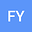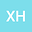••We apply the continuation theorem of Mawhin to ensure that a second-order nonlinear difference equation of the form $$\Delta^2 u(k-1) +a(k)u^{n}(k)-b(k)u^{n+1}(k)+c(k)u^{n+2}(k)=0$$ with periodic boundary conditions possesses at least two nontrivial positive solutions, where $n>0$ is a finite positive integer, $\Delta u(k)=u(k+1)-u(k)$ is the forward difference operator and $a(k),b(k),c(k)$ are $T$-periodic functions on $\mathbb{R}$.